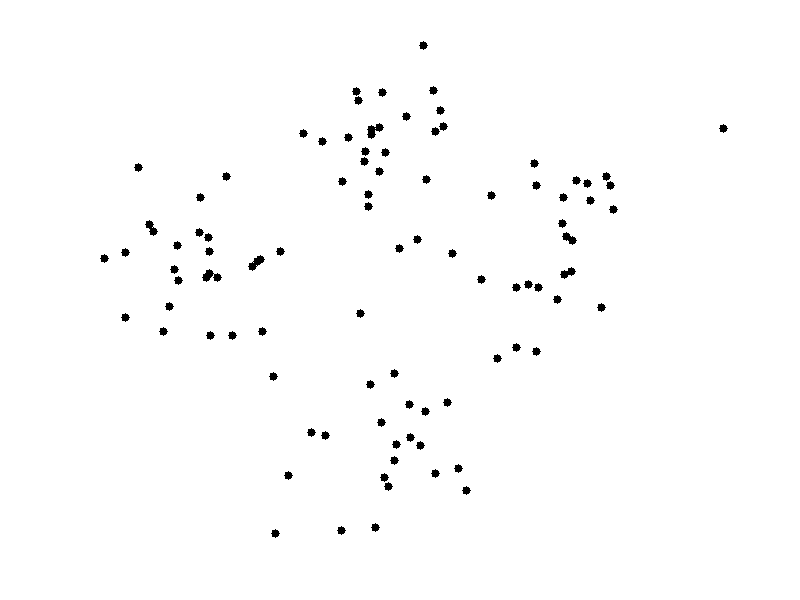Posts tagged with: k-means

## How K-means clustering works

K-means is the most primitive and easy to use clustering algorithm (also a Machine Learning algorithm).
There are 4 basic steps of K-means:

1. Choose K different initial data points on instance space (as initial centroids) – centroid is the mean points of the clusters that overview the attributes of the classes-.
2. Assign each object to the nearest centroid.
3. After all the object are assigned, recalculate the centroids by taking the averages of the current classes (clusters)
4. Do 2-3 until centroid are stabilized.

Caveats for K-means:

• Although it can be proved that the procedure will always terminate, the k-means algorithm does not necessarily find the most optimal configuration, corresponding to the global objective function minimum.
• The algorithm is also significantly sensitive to the initial randomly selected cluster centres. The k-means algorithm can be run multiple times to reduce this effect.

Here is the basic animation to show the intuition of K-means.